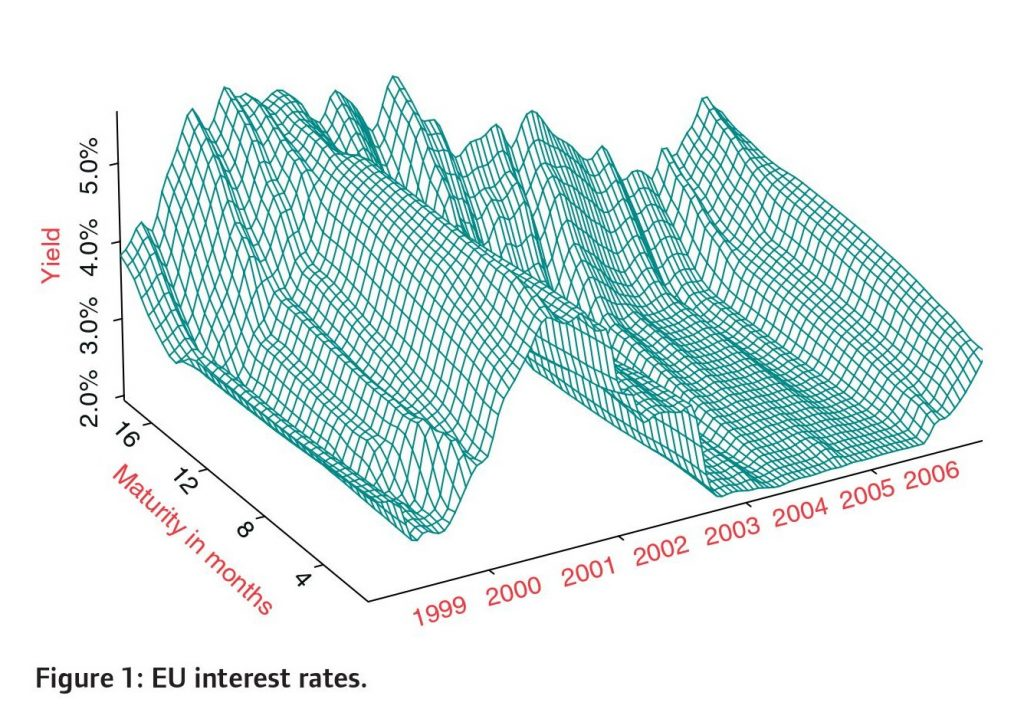You might be interested in...

# Forecasting the Yield Curve with S-Plus

## Dario Cziraky shows how to implement Nelson-Siegel and Svensson models using non-linear least squares and how to obtain standard errors and confidence intervals for the parametersMethods capable of forecasting the entire yield curve based on a time series extension of the Nelson-Siegel model Nelson and Siegel (1987) were suggested in the literature and compared to the non-parametric alternatives Diebold and Li (2004). While relatively successful in forecasting the yield curve, the Nelson-Siegel model tends to have poor fit to highly nonlinear yield curves and at the long end of the term structure, although this can be improved by considering the Svensson (1994) model. However, we find that Nelson-Siegel and Svensson models have poor forecasting performance around the points of non-parallel shifts, hence making them potentially problematic in interest rate risk management.

In this paper we show how to implement these models using non-linear least squares and how to obtain standard errors and confidence intervals for the parameters, which proves to be useful in assessing the goodness-of-fit at specific points in the term structure, such as at the events of non-parallel shifts. Furthermore, we consider an alternative model based on principal components and smoothing splines, which gives improved forecasting performance, particularly for the highly non-linear changes in the term structure curvature.

For empirical illustrations we use the Euribor short-term interest rates with maturities ranging from one week to one year and the Government bond yields with longer maturities of 2 to 10 years, which gives a fairly complete European term structure data (Figure 1). Considering a widely spread term structure presents a modelling challenge, especially when attempting to forecast the yield curve. The EU term structure has notably changed both slope and curvature at several points from the 2001–2006 period covered by our data. Several of the changes can be viewed as non-parallel shifts in the term structure, hence causing non-linear shifts in the shape of the yield curve at a number of time points.

In this paper we carry out an array of backtests comparing out-of sample performance of various multivariate linear models such as PCAVAR and multivariate GARCH models, along with a combinations of Nelson-Siegel-Svensson and MGARCH methods. We adopt a hands-on approach and demonstrating how the suggested methods can be easily implemented in S or R programming languages, illustrating the methods on the EU terms structure data.130322_cziraky